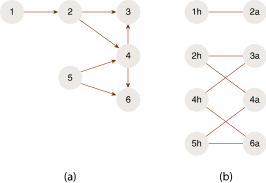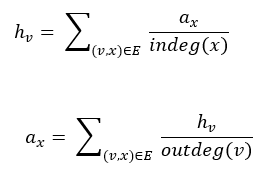# 1.1 - 8.10 - SALSA - Teradata Vantage

## Teradata Vantage™ - Machine Learning Engine Analytic Function Reference

Product
Release Number
1.1
8.10
Release Date
October 2019
Content Type
Programming Reference
Publication ID
B700-4003-079K
Language
English (United States)

Stochastic Approach for Link-Structure Analysis (SALSA) is a link analysis algorithm originally developed for evaluating the importance of web pages (similar to the PageRank algorithm).

In SALSA, a collection of web pages is transformed into a bipartite graph:

`G=(V,X,E)

where

{νV} are hub vertices located on the left side of the graph.

{xX} are authority vertices located on the right side of the graph.

{(ν,x) Î E} are edges linking the hub vertices and authority vertices.

The following figure shows an example on a bipartite graph, which shows the transforming (a) of the collection into (b) bipartite `G.For each hub vertex in the graph, SALSA computes a hub score (h ν ), and for each authority vertex, an authority score (h x) is associated with. The hub/authority score is defined by analyzing a random walk on the bipartite graph, wherein the steps from hub vertex to authority vertex (from the left side of the bipartite graph to the right side) are called forward steps, and the steps from the authority vertex to the hub vertex are called backward steps.

The hub score (h ν ) is defined as the probability of visiting the hub vertex ν and the authority score (a x ) is the probability of visiting the authority vertex x in a random walk on the bipartite graph.

The hub score (h ν ) and authority score (a x ) can be computed using this update rule until convergence: